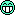programming forums Java Mobile Certification Databases Caching Books Engineering Micro Controllers OS Languages Paradigms IDEs Build Tools Frameworks Application Servers Open Source This Site Careers Other Pie Elite all forums
this forum made possible by our volunteer staff, including ...
Marshals:
• Campbell Ritchie
• Paul Clapham
• Ron McLeod
• Jeanne Boyarsky
• Tim Cooke
Sheriffs:
• Liutauras Vilda
• paul wheaton
• Henry Wong
Saloon Keepers:
• Tim Moores
• Tim Holloway
• Stephan van Hulst
• Carey Brown
• Frits Walraven
Bartenders:
• Piet Souris
• Himai Minh

# Strings

Greenhorn
Posts: 23
••Number of slices to send:
Optional 'thank-you' note:
••Dear All,
I found it confusing with the following code.I have commented the results i expected, but its giving "false" for all comparisons[just i'm checking only for references]
-------------------------My Assumptions--------------------------
Note: Case# = c#
In c1, s1 creates a new string object & s2, while trying to create a new string object, checks for the same literal & if its present should use the same.So, i opted "true".Also, seeing the result, "false", I assumed that by Java specifications definition , whenever a string is created using "=" a new String is created.Am i right?
In c2, the answer should be "true', but, its "false".lllrly for c6.
Request clarification
-------------------------My Assumptions Ends---------------------
class A1
{
public static void main(String arf[])
{
String s1 = "A";
String s2 = "A";
String s3 = "C";
String s4 = s3;
String s5 = "D";
String s6 = new String("D");
String s7 = new String("E");
String s8 = new String("E");
String s9 = new String("F");
String s10 = "F";
String s11 = new String("H");
String s12 = s11;
/*c1*/ System.out.println("s1 == s2 ==> " + s1 == s2);//true
/*c2*/ System.out.println("s3 == s4 ==> " + s3 == s4);//true
/*c3*/ System.out.println("s5 == s6 ==> " + s5 == s6);//false
/*c4*/ System.out.println("s7 == s8 ==> " + s7 == s8);//false
/*c5*/ System.out.println("s9 == s10 ==> " + s9 == 10);//false
/*c6*/ System.out.println("s11 == s12 ==> " + s11 == s12);//true
}//EoMain
}//EoA1
Thanks & Rgds,
ANaveenS
------------------
ANaveenS

Naveen Arumugam
Greenhorn
Posts: 23
••Number of slices to send:
Optional 'thank-you' note:
••Dear All,
I found the answer, the problem is with operator precedence
ie., "+" has got more precedence than "==" , so 'message string + string1[used for comparison] gets into one string which is then compared to the string2, so evaluates to false. If we introduce a parenthesis, then the problem is solved.

_CODE__________
class BBBBB1{

public static void main(String arf[]){

String s1 = "A";
String s2 = "A";
String s3 = "C";
String s4 = s3;
String s5 = "D";
String s6 = new String("D");
String s7 = new String("E");
String s8 = new String("E");
String s9 = new String("F");
String s10 = "F";
String s11 = new String("H");
String s12 = s11;
String s13,s14;
s13 = new String("H");
s14 = s13;
System.out.println("s1 == s2 ==> " + (s1 == s2)); //true
System.out.println("s3 == s4 ==> " + (s3 == s4)); //true
System.out.println("s5 == s6 ==> " + (s5 == s6)); //false
System.out.println("s7 == s8 ==> " + (s7 == s8)); //false
System.out.println("s9 == s10 ==> " + (s9 == s10));//false
System.out.println("s11 == s12 ==> " + (s11 == s12));//true
System.out.println("s11 == s12 ==> " + (s13 == s14));//true
}
}

Thanks
ANaveenS

Greenhorn
Posts: 18
••Number of slices to send:
Optional 'thank-you' note:
••hi there..
i tried to run ur code..
***************************
class A0324
{
public static void main(String args[])
{
String s1 = "A";
String s2 = "A";
String s3 = "C";
String s4 = s3;
String s5 = "D";
String s6 = new String("D");
String s7 = new String("E");
String s8 = new String("E");
String s9 = new String("F");
String s10 = "F";
String s11 = new String("H");
String s12 = s11;
/*c1*/ System.out.println("s1 == s2 ==> " + (s1 == s2));//true
/*c2*/ System.out.println("s3 == s4 ==> " + (s3 == s4));//true
/*c3*/ System.out.println("s5 == s6 ==> " + (s5 == s6));//false
/*c4*/ System.out.println("s7 == s8 ==> " + (s7 == s8));//false
/*c5*/ System.out.println("s9 == s10 ==> " +( s9 == s10));//false
/*c6*/ System.out.println("s11 == s12 ==> " + (s11 ==s12));//true
}//EoMain
}//EoA1
************************************
output:
s1==s2==>true
s3==s4==>true
s5==s6==>false
s7==s8==>false
s9==s10==>false
s11==s12==>true
*******************************************
String is immutable.. always remember that.
also, ur code got some error..
* main(String args[ ]) --> not arf[ ]
*System.out.println("s1==s2==>" + (s1==s2));
--> not just "s1==s2==>"+s1==s2
its look like: "s1==s2==>+s1"==s2 // always gets false
hope this help. good weekend.(try it~)
^__^*
martha

Martha Yeh
Greenhorn
Posts: 18
••Number of slices to send:
Optional 'thank-you' note:
••the arf[ ] work fine tooforgive me.. sleepy time here.. (12:41am)
goodu got the point.. good weekend again.
martha

Naveen Arumugam
Greenhorn
Posts: 23
••Number of slices to send:
Optional 'thank-you' note:
••Martha